Previous: Compressible Mathematical Model Up: TCFD Solvers Next: Unstructured Grid
This is an automatically generated documentation by LaTeX2HTML utility. In case of any issue, please, contact us at info@cfdsupport.com.

## Incompressible Mathematical Model

Incompressible model solves the system of incompressible Navier-Stokes equations,.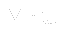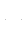0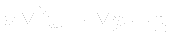• Mass conservation• Momentum conservation• where: Symbol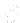is partial derivative,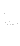is velocity vector,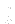is time,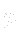is static pressure [    Pa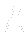kgm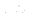m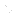s],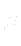is dynamic viscosity,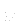is kinematic viscosity and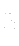is gravitational acceleration vector.
• The whole system is closed with boundary conditions.